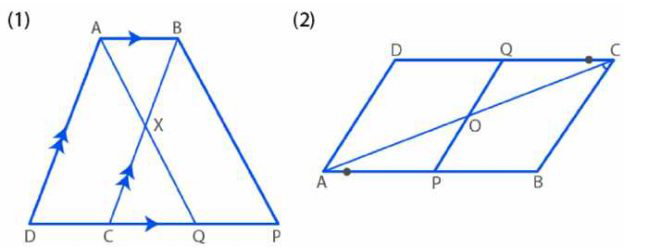# ML Aggarwal Solutions Class 9 Mathematics Solutions for Rectilinear Figures Exercise 13.1 in Chapter 13 - Rectilinear Figures

(a) In figure (1) given below, ABCD is a parallelogram and X is the mid-point of BC. The line AX

produced meets DC produced at Q. The parallelogram ABPQ is completed.

Prove that:

(i) the triangles ABX and QCX are congruent;

(ii)DC = CQ = QP

(b) In figure (2) given below, points P and Q have been taken on opposite sides AB and CD

respectively of a parallelogram ABCD such that AP = CQ. Show that AC and PQ bisect each

other.(a) Given: ABCD is parallelogram and X is mid-point of BC. The line AX produced meets DC produced

at Q and ABPQ is a || gm.

To prove: (i) ∆ABX ≅ ∆QCX

(ii) DC = CQ = QP

Proof:

In ∆ABX and ∆QCX, we have

BX = XC [X is the mid-point of BC]

∠AXB = ∠CXQ [Vertically opposite angles]

∠XCQ = ∠XBA [Alternate angle, since AB || CQ]

So, ABX ≅ ∆QCX by A.S.A axiom of congruence

Now, by C.P.C.T

CQ = AB

But,

AB = DC and AB = QP [As ABCD and ABPQ are || gms]

Hence,

DC = CQ = QP

(b) In || gm ABCD, P and Q are points on AB and CD respectively, PQ and AC intersect each other at O

and AP = CQ

To prove: AC and PQ bisect each other i.e. AO = OC and PO = OQ

Proof:

In ∆AOP and ∆COQ

AP = CQ [Given]

∠AOP = ∠COQ [Vertically opposite angles]

∠OAP = ∠OCP [Alternate angles]

So, ∆AOP ≅ ∆COQ by A.A.S axiom of congruence

Now, by C.P.C.T

OP = OQ and OA = OC

Hence proved.

Related Questions

Lido

Courses

Teachers

Book a Demo with us

Syllabus

Maths
CBSE
Maths
ICSE
Science
CBSE

Science
ICSE
English
CBSE
English
ICSE
Coding

Terms & Policies

Selina Question Bank

Maths
Physics
Biology

Allied Question Bank

Chemistry
Connect with us on social media!